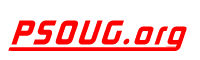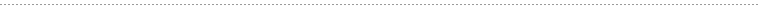Quick Search:# Term: SUM

Definition:
In Oracle PL/SQL, SUM is a built in function which can be used to sum or total the values of a column. It can be used for both aggregate and analytic functions. SUM takes a numeric input and returns numeric output as the sum of all values contained by the column.

Example Syntax:

Aggregate Usage:

`SUM(expression)`

Analytic Usage:

`SUM([DISTINCT | ALL] expression)  OVER  (partition clause)`

Example Usage:

Aggregate Use
The SQL query below displays total salary of all employees in each department.

```SELECT DEPT, SUM(SAL) SUM_SAL
FROM EMPLOYEE
GROUP_BY DEPT
ORDER_BY DEPT;

DEPT    SUM_SAL
---------- ----------
10       4800
20      14100
30       9400
40       6400
50       3200/```

Analytic Use
The query above can be reframed in order to demonstrate the analytic behavior of the SUM function:

```SELECT DISTINCT DEPT, SUM(SAL)  OVER (PARTITION BY DEPT ORDER_BY DEPT) SUM_SAL
FROM EMPLOYEE
ORDER_BY DEPT;

DEPT    SUM_SAL
---------- ----------
10       4800
20      14100
30       9400
40       6400
50       3200```